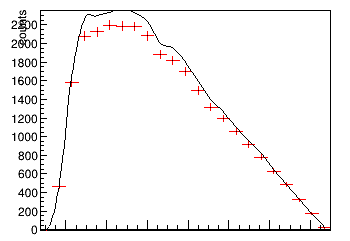# Explicitly set error for TRatio plot

Hi all, I am trying TRatioPlot recently, where I followed steps from ROOT: TRatioPlot Class Reference.
However it seems error bars is plotted for h1. What if I want to plot h2/h1 (reverse the arguments to `TRatioPlot(h2,h1)`), with the error bars for h1. Is there any method to explicitly set the plot style ? (something like `HIST C` options, but for individual histogram in the TRatioPlot)

``````void ratioplot1() {
gStyle->SetOptStat(0);
auto c1 = new TCanvas("c1", "A ratio example");
auto h1 = new TH1D("h1", "h1", 50, 0, 10);
auto h2 = new TH1D("h2", "h2", 50, 0, 10);
auto f1 = new TF1("f1", "exp(- x/ )");
f1->SetParameter(0, 3);
h1->FillRandom("f1", 1900);
h2->FillRandom("f1", 2000);
h1->Sumw2();
h2->Scale(1.9 / 2.);
h1->GetXaxis()->SetTitle("x");
h1->GetYaxis()->SetTitle("y");
auto rp = new TRatioPlot(h1, h2);
c1->SetTicks(0, 1);
rp->Draw();
rp->GetLowYaxis()->SetNdivisions(505);
}
``````

_ROOT Version: 6.24 (PyROOT via conda)
_Platform:Centos7
_Compiler: gcc9

``````auto rp = new TRatioPlot(h2, h1);
rp->SetH1DrawOpt("E");
rp->SetH2DrawOpt("HIST C");
``````
1 Like

I have another question for you:
How to set auto scale on Y-axis for this TRatio (It can be done automatically with THStack, but how about for the TRatio) ?

I would suggest you put the histogram with the largest maximum in:

``````auto rp = new TRatioPlot(h1, h2);
``````

That’s exactly my workaround before the post, and it produced an truncated plot like this:However, what I want to achieve is a plot (lower peak histo) / (higher peak histo) that without being cut at the highest peak (on the upper panel) so I could get a ratio that is in [0,1].

If it is just about the plot, try:

``````rp->Draw(); // c1->Modified(); c1->Update();
rp->GetUpperRefYaxis()->SetRangeUser(0., 2500.);
``````
1 Like

Or change the maximum of the 1st histogram

1 Like

Did you mean `h1->SetMaximum(3000);` ?

Yes for instance.

1 Like